# Are you a mod or a rocker?

This post was supposed to go up just a few days after the last one, but stuff got in the way. At least we’re in the same month.

Shortly after that post went up, I got an email from reader Jason Reene.

When I plug “tan^-1(cot(x))” into my TI-89 (still my go-to quick symbolic algebra tool) it returns “mod(-x, π) - π/2”

It took me a few minutes to convince myself that was an equivalent result.

It took me a lot longer to convince myself that it was equivalent, partly because I wasn’t sure how the TI-89 handles the mod function when the dividend is negative and the divisor is positive, but mostly because I was having a hard time figuring out which quadrant mod(-x, n) would be in for a given quadrant of x.

But it does work, even for angles well outside the (0, π/2) domain that my problem was restricted to. Here’s Mathematica’s plot of ArcTan[Cot[x]] over (-π, π):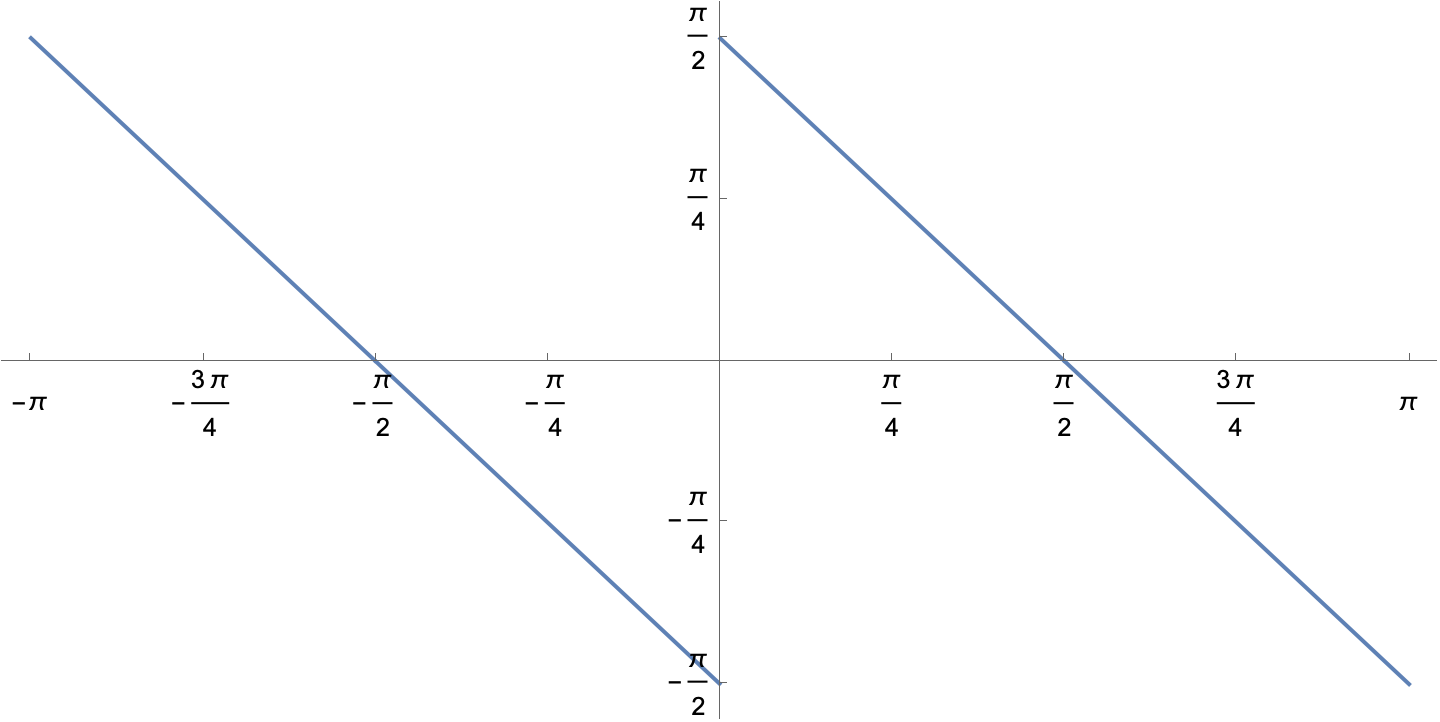And here’s its plot of Mod[-x, Pi] - Pi/2 over the same domain: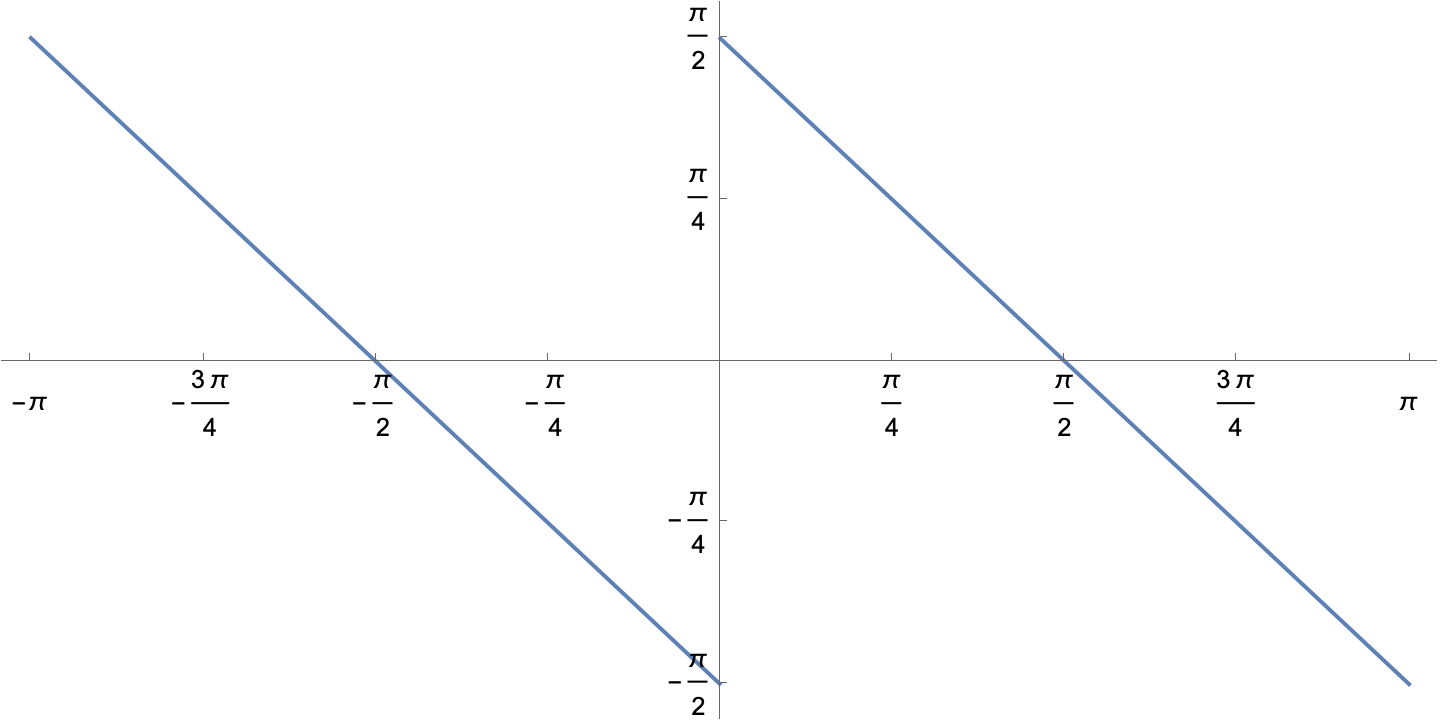Apparently, Mathematica treats the Mod function the same way Jason’s TI-89 does. Not all programming languages do.

After making these plots, I started thinking about the tangent, inverse tangent, and modulo functions, and how their definitions could easily change the answers Jason and I got.

Let’s start with tangent and its inverse. Here’s the tangent plotted over a decent range of angles: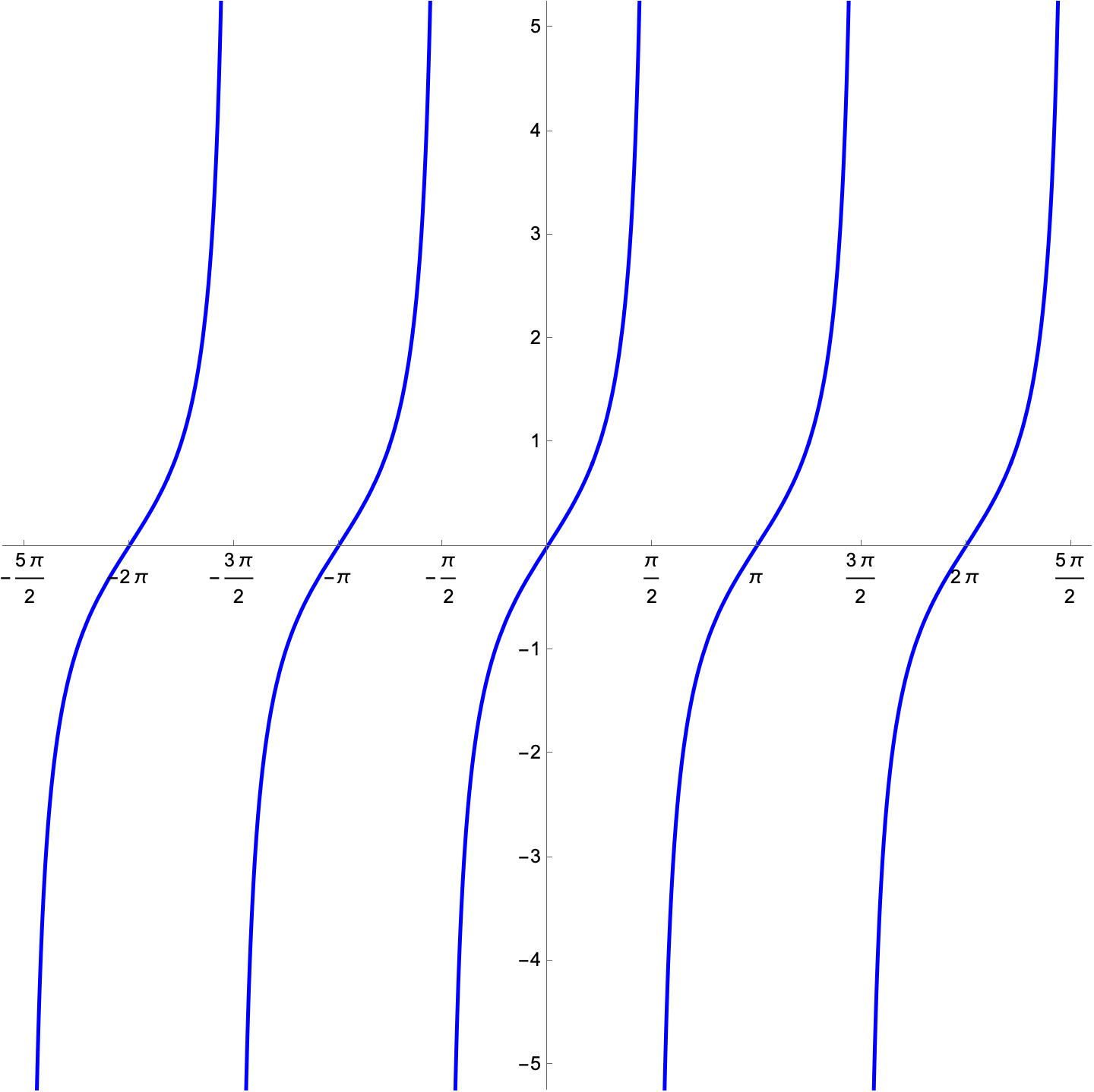To see its inverse, we exchange the horizontal and vertical axes: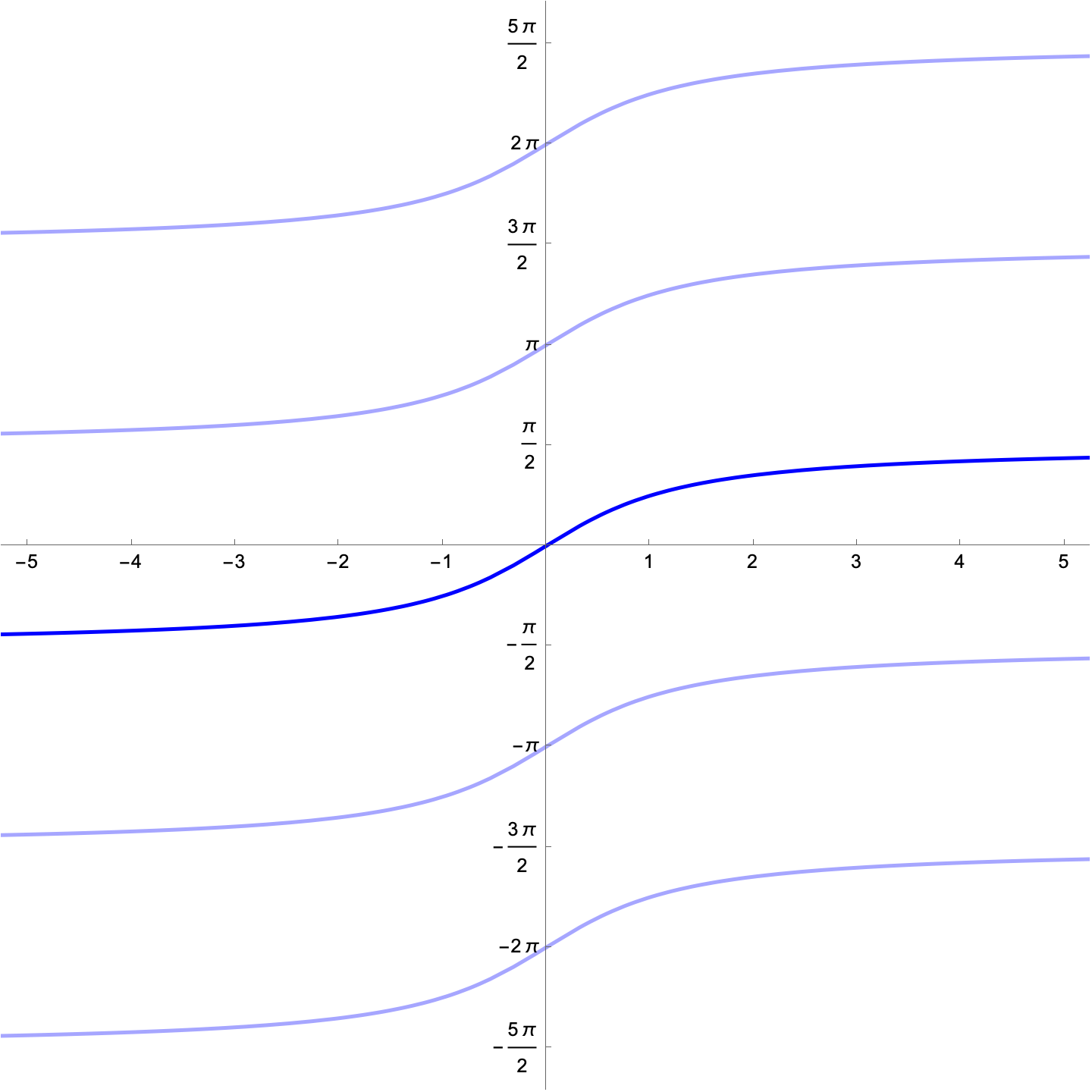Because this has multiple values for every argument, we have to choose which one our inverse tangent function will return. This is called the principal value. As far as I know, every programming language and every calculator chooses the one I’ve made a darker blue—the one that returns a value between -π/2 and π/2. So if you ask your calculator for the inverse tangent of a positive number, it gives you an angle in the first quadrant; if you ask it to give you the inverse tangent of a negative number, it gives you an angle in the fourth quadrant.

And if you’ve forgotten what that “quadrant” stuff is, this should refresh your memory. The arrows point in the positive directions.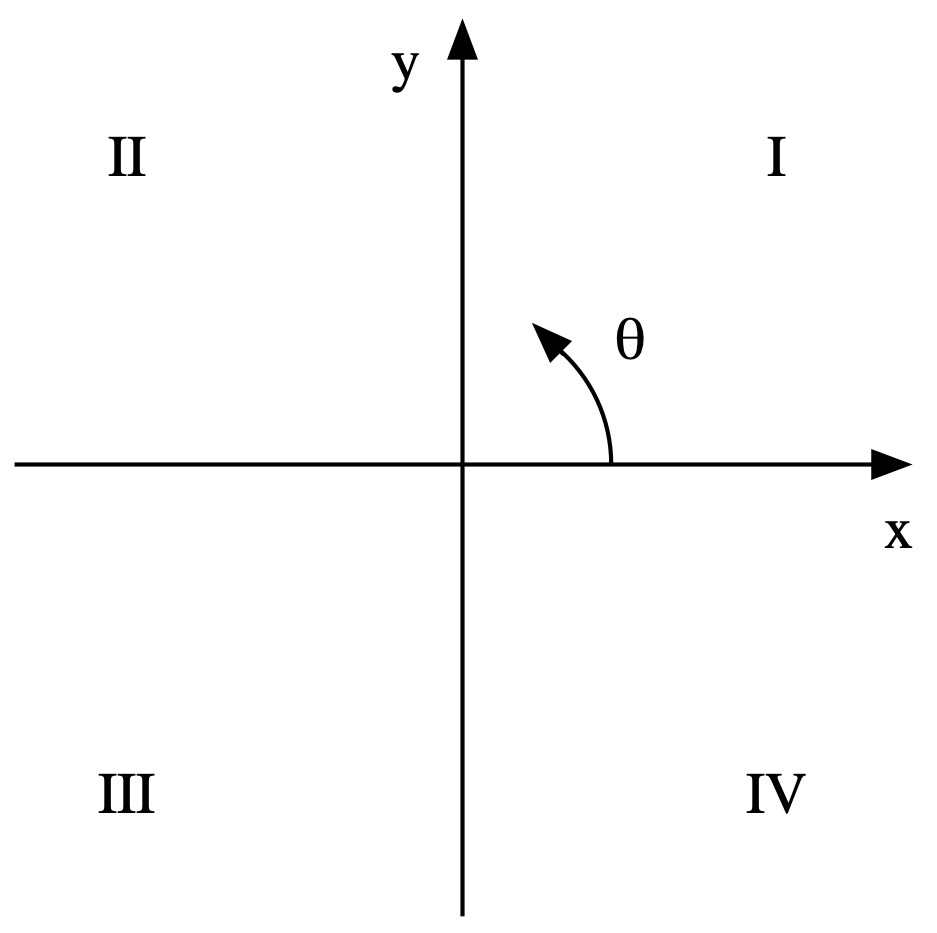Let’s move on to modulo. This is basically what you learned as “remainder” when you were first doing division. And when you’re dealing with positive integers only, modulo is exactly what you learned back then. Extending modulo to non-integer numbers is straightforward, but the tricky bits come when either the dividend (the number you’re dividing) or the divisor (the number you’re dividing it by) are negative.

For example, if you want -7 mod 3, you could think of it as

7 = 3 × (-2) – 1


so -7 mod 3 would be –1. Or you could think of it as

7 = 3 × (-3) + 2


and -7 mod 3 would be 2. Both answers are valid, but if you’re designing a programming language or a calculator, you have to choose one or the other. For this problem, Perl, Python, and Ruby return 2, while AppleScript and JavaScript return -1.

There’s a lot more to modulo—we haven’t discussed the divisor being negative—but that’s enough for our purposes. You can look up the various definitions on Wikipedia.

When the dividend is negative and the divisor positive, both Mathematica and the TI-89 return a positive result, which is why my Mod graph above (made in Mathematica) and the result from Jason’s calculator agree.

To show how tricky the TI-89’s formula is, let’s see how it transforms an angle in the first quadrant. We’ll use π/6, the same angle we converted with the ArcTan[Cot[θ]] formula in the last post. You can see the manipulations graphically in this image: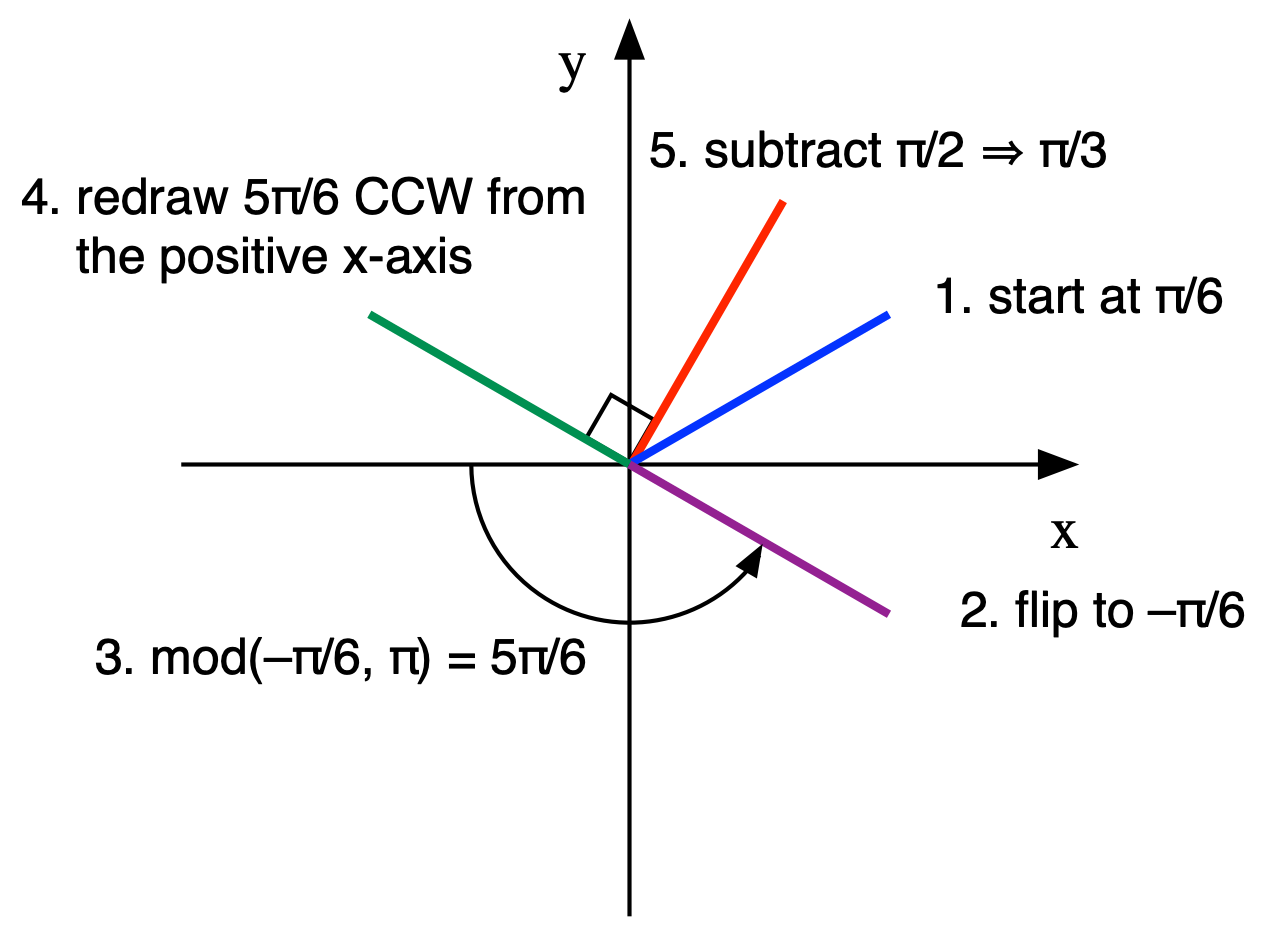The hardest part to visualize is, of course, the modulo operation. It’s the smallest counterclockwise angle from an integer multiple of π to the purple line that was drawn in Step 2. In this case, the multiple of π we use is –π, the negative x-axis, and the CCW angle to the purple line is 5π/6.

As you can see, we do get to π/3, just not as easily as we did in the last post, where we just took the complement of the angle. On the other hand, this modulo formula works for angles outside of the first quadrant, and Jason’s TI-89 came up with it on its own. As you recall, Mathematica got stuck on ArcTan[Cot[θ]] and wouldn’t reduce it further. We had to figure out that it was the complement of π ourselves.

Thanks to Jason for an interesting view on this problem, and congratulations to the computer algebra people at Texas Instruments for a clever solution.